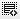## can you please explain HLOOKUP also?0
please explain the function of vlookup and hlookup0

All Lookup functions are used to lookup information in a database. There are 2 kinds of lookup functions.
1) Vlookup Function
2) Hlookup Function

Vlookup Function - Looks up the data vertically down a particular column. we use this function when our comparison values are located in a column to the left data you want to find.

Syntax:

=VLOOKUP (Lookup_Value, Table_Array, Column_Index, Lookup_Type)

Hlookup Function - Looks up the data horizontally across a particular row. we use this function when comparison values are located in a row across the top of the table and you want to look down a specified number of rows.

Syntax:
=HLOOKUP (Lookup_Value, Table_Array, Row_Index, Lookup_Type)0

The Microsoft Excel HLOOKUP function performs a horizontal lookup by searching for a value in the top row of table_array and returning the value in the same column based on the index_number.0

The Microsoft Excel HLOOKUP function performs a horizontal lookup by searching for a value in the top row of table_array and returning the value in the same column based on the index_number

Click on thisicon to add code snippet.